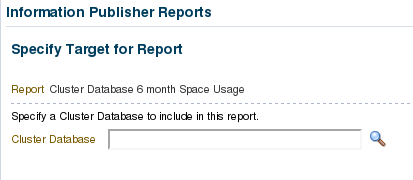# OEM Report: Last 6 month Database Space Usage and Growth

Hello All!
So I had worked in some very useful reports to have in OEM. In next weeks I’ll share some code you may like… 🙂

To create it? Enterprise -> Reports -> Information Reports. There are several nice default reports there you me like.

Now, let’s go to the first Report, as per title:

1. First you Select the Database:2. Then you see the report:That’s nice, right?
Here is a report for another database with actual 640GB average growth per month (its expected, once DBSize is over 60TB):So, Mat, can you share the queries? Of course:

# First Table (Database Space usage at the end of the month in the last 6 months):

```SELECT SPACE_ALLOCATED_MONTHLY.target_name  as "Database Name",
SPACE_ALLOCATED_MONTHLY.rtime as "Last Day of Month",
round(SPACE_ALLOCATED_MONTHLY.allocated,2) as  "Space Allocated",
round(SPACE_USED_MONTLY.useds,2) as "Used Space",
round((SPACE_ALLOCATED_MONTHLY.allocated-SPACE_USED_MONTLY.useds),2) as "Free Space",
round(((SPACE_USED_MONTLY.useds/SPACE_ALLOCATED_MONTHLY.allocated)*100),2) as "Used %"
from
(select T.TARGET_NAME as target_name, --SPACE ALLOCATED MONTHLY
M.ROLLUP_TIMESTAMP  as rtime,
sum(M.AVERAGE/1024) as allocated
from   MGMT\$METRIC_DAILY M,
MGMT\$TARGET_TYPE T,
(select T.TARGET_NAME target_name,
extract (year from m.rollup_timestamp) tyear,
extract (month from m.rollup_timestamp) tmonth,
max(extract (day from m.rollup_timestamp)) maxday
from   MGMT\$METRIC_DAILY M,
MGMT\$TARGET_TYPE T where
T.TARGET_GUID=hextoraw(??EMIP_BIND_TARGET_GUID??)  and
(T.TARGET_TYPE='rac_database' OR
(T.TARGET_TYPE='oracle_database' and T.TYPE_QUALIFIER3 != 'RACINST')) and
M.TARGET_GUID=T.TARGET_GUID and
M.METRIC_GUID=T.METRIC_GUID and
T.METRIC_NAME='tbspAllocation' and
T.METRIC_COLUMN='spaceAllocated' and
M.METRIC_NAME=T.METRIC_NAME and
M.METRIC_COLUMN=T.METRIC_COLUMN
and  M.ROLLUP_TIMESTAMP >= to_timestamp(trunc((sysdate-180),'MM'))
group by t.target_name, extract (year from m.rollup_timestamp),extract (month from m.rollup_timestamp)) last_day_of_month
where    T.TARGET_GUID=hextoraw(??EMIP_BIND_TARGET_GUID??)  and
(T.TARGET_TYPE='rac_database' OR
(T.TARGET_TYPE='oracle_database' and T.TYPE_QUALIFIER3 != 'RACINST')) and
M.TARGET_GUID=T.TARGET_GUID and
M.METRIC_GUID=T.METRIC_GUID and
T.METRIC_NAME='tbspAllocation' and
T.METRIC_COLUMN='spaceAllocated' and
M.METRIC_NAME=T.METRIC_NAME and
M.METRIC_COLUMN=T.METRIC_COLUMN
and  M.ROLLUP_TIMESTAMP >= to_timestamp(trunc((sysdate-180),'MM'))
and extract (year from m.rollup_timestamp)=last_day_of_month.tyear
and extract (month from m.rollup_timestamp)=last_day_of_month.tmonth
and extract (day from m.rollup_timestamp)=last_day_of_month.maxday
group by T.TARGET_NAME, M.ROLLUP_TIMESTAMP) SPACE_ALLOCATED_MONTHLY, --SPACE ALLOCATED MONTHLY
(select T.TARGET_NAME target_name,--SPACE USED MONTLY
M.ROLLUP_TIMESTAMP as rtime,
sum(M.AVERAGE/1024) as useds
from   MGMT\$METRIC_DAILY M,
MGMT\$TARGET_TYPE T,
(select T.TARGET_NAME target_name,
extract (year from m.rollup_timestamp) tyear,
extract (month from m.rollup_timestamp) tmonth,
max(extract (day from m.rollup_timestamp)) maxday
from   MGMT\$METRIC_DAILY M,
MGMT\$TARGET_TYPE T where
T.TARGET_GUID=hextoraw(??EMIP_BIND_TARGET_GUID??)  and
(T.TARGET_TYPE='rac_database' OR
(T.TARGET_TYPE='oracle_database' and T.TYPE_QUALIFIER3 != 'RACINST')) and
M.TARGET_GUID=T.TARGET_GUID and
M.METRIC_GUID=T.METRIC_GUID and
T.METRIC_NAME='tbspAllocation' and
T.METRIC_COLUMN='spaceUsed' and
M.METRIC_NAME=T.METRIC_NAME and
M.METRIC_COLUMN=T.METRIC_COLUMN
and  M.ROLLUP_TIMESTAMP >= to_timestamp(trunc((sysdate-180),'MM'))
group by t.target_name, extract (year from m.rollup_timestamp),extract (month from m.rollup_timestamp)) last_day_of_month
where  T.TARGET_GUID=hextoraw(??EMIP_BIND_TARGET_GUID??)  and
(T.TARGET_TYPE='rac_database' OR
(T.TARGET_TYPE='oracle_database' and T.TYPE_QUALIFIER3 != 'RACINST')) and
M.TARGET_GUID=T.TARGET_GUID and
M.METRIC_GUID=T.METRIC_GUID and
T.METRIC_NAME='tbspAllocation' and
T.METRIC_COLUMN='spaceUsed' and
M.METRIC_NAME=T.METRIC_NAME and
M.METRIC_COLUMN=T.METRIC_COLUMN
and  M.ROLLUP_TIMESTAMP >= to_timestamp(trunc((sysdate-180),'MM'))
and extract (year from m.rollup_timestamp)=last_day_of_month.tyear
and extract (month from m.rollup_timestamp)=last_day_of_month.tmonth
and extract (day from m.rollup_timestamp)=last_day_of_month.maxday
group by T.TARGET_NAME, M.ROLLUP_TIMESTAMP) SPACE_USED_MONTLY --SPACE USED MONTLY
where SPACE_USED_MONTLY.target_name=SPACE_ALLOCATED_MONTHLY.target_name
and  SPACE_USED_MONTLY.rtime=SPACE_ALLOCATED_MONTHLY.rtime
order by 2```

# Second Table (General Database Space Usage Information)

```select CURRENT_USEDSIZE.TARGET_NAME "Database Name",
round(MAX_ALLOCATED.SIZE_GB,2) as "6 Month Max Allocation",
round((CURRENT_USEDSIZE.SIZE_GB-FIRST_USEDSPACE.SIZE_GB)/6,2) as "6 Month Avg Monthly Growth"
from
(select T.TARGET_NAME,  --FIRST USED SPACE
M.ROLLUP_TIMESTAMP as MONTH_TIMESTAMP,
sum(M.AVERAGE/1024)as SIZE_GB
from   MGMT\$METRIC_DAILY M,
MGMT\$TARGET_TYPE T,
(select T.TARGET_NAME, MIN(ROLLUP_TIMESTAMP) MIN_TIME
from  MGMT\$METRIC_DAILY M, MGMT\$TARGET_TYPE T
where T.TARGET_GUID=hextoraw(??EMIP_BIND_TARGET_GUID??) and
(T.TARGET_TYPE='rac_database' OR
(T.TARGET_TYPE='oracle_database' and T.TYPE_QUALIFIER3 != 'RACINST')) and
M.TARGET_GUID=T.TARGET_GUID and
M.METRIC_GUID=T.METRIC_GUID and
T.METRIC_NAME='tbspAllocation' and
T.METRIC_COLUMN='spaceUsed' and
M.METRIC_NAME=T.METRIC_NAME and
M.METRIC_COLUMN=T.METRIC_COLUMN and
M.ROLLUP_TIMESTAMP >= to_timestamp(trunc((sysdate-180),'MM')) and
M.ROLLUP_TIMESTAMP <= to_timestamp(sysdate) 			group by T.TARGET_NAME, 			M.METRIC_COLUMN) MIN_DATE 		where T.TARGET_GUID=hextoraw(??EMIP_BIND_TARGET_GUID??) and 		(T.TARGET_TYPE='rac_database' OR  		(T.TARGET_TYPE='oracle_database' and T.TYPE_QUALIFIER3 != 'RACINST')) and       		M.TARGET_GUID=T.TARGET_GUID and 		M.METRIC_GUID=T.METRIC_GUID and 		T.METRIC_NAME='tbspAllocation' and 		T.METRIC_COLUMN='spaceUsed' and 		M.METRIC_NAME=T.METRIC_NAME and 		M.METRIC_COLUMN=T.METRIC_COLUMN and 		T.TARGET_NAME=MIN_DATE.TARGET_NAME AND M.ROLLUP_TIMESTAMP=MIN_DATE.MIN_TIME 		group by t.target_name,m.rollup_timestamp) FIRST_USEDSPACE,	--FIRST USED SPACE 		(select A_SIZE.TARGET_NAME, --MAX ALLOCATED SPACE IN 6 MONTHS 		round(max(A_SIZE.SIZE_GB),2) as SIZE_GB 		from (select T.TARGET_NAME,   			M.ROLLUP_TIMESTAMP as MONTH_TIMESTAMP, 			sum(M.AVERAGE/1024) as SIZE_GB 			from MGMT\$METRIC_DAILY M, 			MGMT\$TARGET_TYPE T 			where T.TARGET_GUID=hextoraw(??EMIP_BIND_TARGET_GUID??) and  			(T.TARGET_TYPE='rac_database' OR  			(T.TARGET_TYPE='oracle_database' and T.TYPE_QUALIFIER3 != 'RACINST')) and       			M.TARGET_GUID=T.TARGET_GUID and 			M.METRIC_GUID=T.METRIC_GUID and 			T.METRIC_NAME='tbspAllocation' and 			T.METRIC_COLUMN='spaceAllocated' and 			M.ROLLUP_TIMESTAMP >= to_timestamp(trunc((sysdate-180),'MM')) and
M.ROLLUP_TIMESTAMP <= to_timestamp(sysdate) 			group by T.TARGET_NAME, 			M.METRIC_COLUMN, 			M.ROLLUP_TIMESTAMP) A_SIZE 		group by A_SIZE.TARGET_NAME) MAX_ALLOCATED,	--MAX ALLOCATED SPACE IN 6 MONTHS				   		(select T.TARGET_NAME,   --CURRENT USED SPACE 		M.ROLLUP_TIMESTAMP as MONTH_TIMESTAMP, 		sum(M.AVERAGE/1024)as SIZE_GB 		from   MGMT\$METRIC_DAILY M, 		MGMT\$TARGET_TYPE T, 			(select T.TARGET_NAME, max(ROLLUP_TIMESTAMP) MAX_TIME  			from  MGMT\$METRIC_DAILY M, MGMT\$TARGET_TYPE T	   			where T.TARGET_GUID=hextoraw(??EMIP_BIND_TARGET_GUID??) and 			(T.TARGET_TYPE='rac_database' OR  			(T.TARGET_TYPE='oracle_database' and T.TYPE_QUALIFIER3 != 'RACINST')) and      	 			M.TARGET_GUID=T.TARGET_GUID and 			M.METRIC_GUID=T.METRIC_GUID and 			T.METRIC_NAME='tbspAllocation' and 			T.METRIC_COLUMN='spaceUsed' and 			M.METRIC_NAME=T.METRIC_NAME and 			M.METRIC_COLUMN=T.METRIC_COLUMN and M.ROLLUP_TIMESTAMP >= to_timestamp(trunc((sysdate-180),'MM')) and
M.ROLLUP_TIMESTAMP <= to_timestamp(sysdate)
group by T.TARGET_NAME,
M.METRIC_COLUMN) MAX_DATE
where T.TARGET_GUID=hextoraw(??EMIP_BIND_TARGET_GUID??) and
(T.TARGET_TYPE='rac_database' OR
(T.TARGET_TYPE='oracle_database' and T.TYPE_QUALIFIER3 != 'RACINST')) and
M.TARGET_GUID=T.TARGET_GUID and
M.METRIC_GUID=T.METRIC_GUID and
T.METRIC_NAME='tbspAllocation' and
T.METRIC_COLUMN='spaceUsed' and
M.METRIC_NAME=T.METRIC_NAME and
M.METRIC_COLUMN=T.METRIC_COLUMN and
T.TARGET_NAME=MAX_DATE.TARGET_NAME AND M.ROLLUP_TIMESTAMP=MAX_DATE.MAX_TIME
group by t.target_name,m.rollup_timestamp) CURRENT_USEDSIZE		--CURRENT USED SPACE
WHERE
CURRENT_USEDSIZE.TARGET_NAME=FIRST_USEDSPACE.TARGET_NAME and
CURRENT_USEDSIZE.TARGET_NAME=MAX_ALLOCATED.TARGET_NAME
order by CURRENT_USEDSIZE.TARGET_NAME```

# Chart (Average of Database Space Usage in the past 6 months)

```select decode(METRIC_COLUMN, 'spaceAllocated', 'Average Allocated(GB)', 'spaceUsed', 'Average Used(GB)'),
MONTH_TIMESTAMP,
avg(VALUE)
from (select M.METRIC_COLUMN as METRIC_COLUMN,
to_date(to_char(M.ROLLUP_TIMESTAMP,'YYYY-MM'), 'YYYY-MM') as MONTH_TIMESTAMP,
sum(M.AVERAGE/1024) as VALUE
from MGMT\$METRIC_DAILY M,
MGMT\$TARGET_TYPE T
where  T.TARGET_GUID=hextoraw(??EMIP_BIND_TARGET_GUID??)
and (T.TARGET_TYPE='rac_database'
or
(T.TARGET_TYPE='oracle_database' and T.TYPE_QUALIFIER3 != 'RACINST'))
and M.TARGET_GUID=T.TARGET_GUID
and M.METRIC_GUID=T.METRIC_GUID
and M.ROLLUP_TIMESTAMP >= to_timestamp(trunc((sysdate-180),'MM'))
and M.ROLLUP_TIMESTAMP <= to_timestamp(sysdate)
and T.METRIC_NAME='tbspAllocation'
and (T.METRIC_COLUMN='spaceAllocated'
or T.METRIC_COLUMN='spaceUsed')
GROUP BY m.metric_column, m.rollup_timestamp)
GROUP BY metric_column, month_timestamp
ORDER BY month_timestamp ASC, metric_column DESC```

Hope you enjoy it.
Cheers!

1.brenda eagleston

can’t use due to the bind variable…

2.Pablo Rubino

Hi, how to replace ??EMIP_BIND_TARGET_GUID??
Regards.

3.charan

how to fix the error mentioned by rajesh?

4.RAJESH

ORA-00911: invalid character
??EMIP_BIND_TARGET_GUID??

1.matheusdba

Hello Rajesh, this is a OEM bind variable.

1.RAJESH

ok it seems that it is bind variable.what to do to fix this issue

This site uses Akismet to reduce spam. Learn how your comment data is processed.